Question

# The electrons in the beam of a television tube have a kinetic energy of 2.40 ×...

The electrons in the beam of a television tube have a kinetic energy of 2.40 × 10-15 J. Initially, the electrons move horizontally from west to east. The vertical component of the earth's magnetic field points down, toward the surface of the earth, and has a magnitude of 1.67 × 10-5 T. What is the acceleration of an electron due to this field component?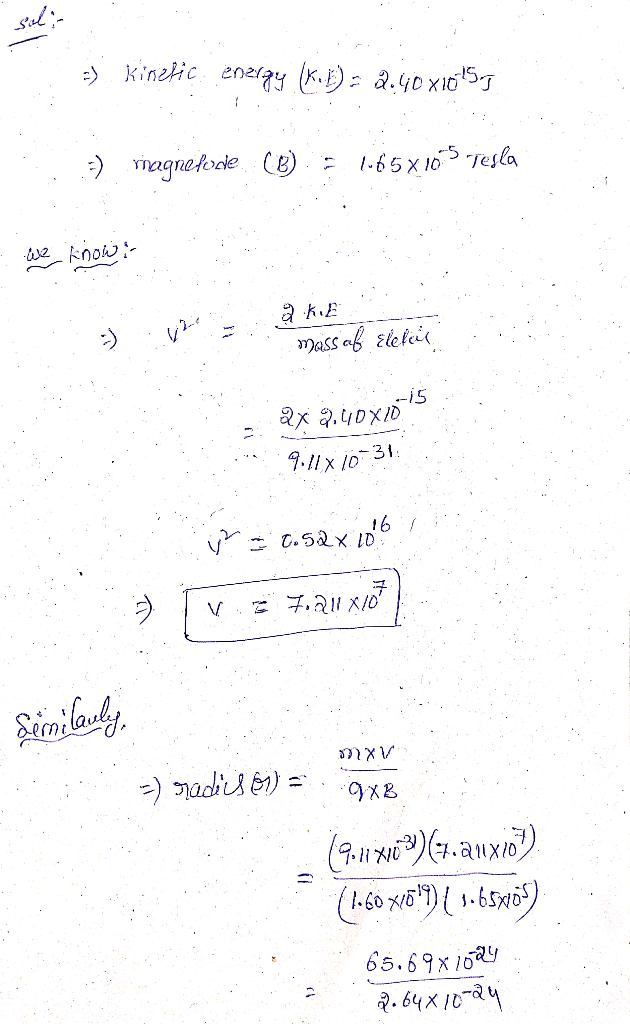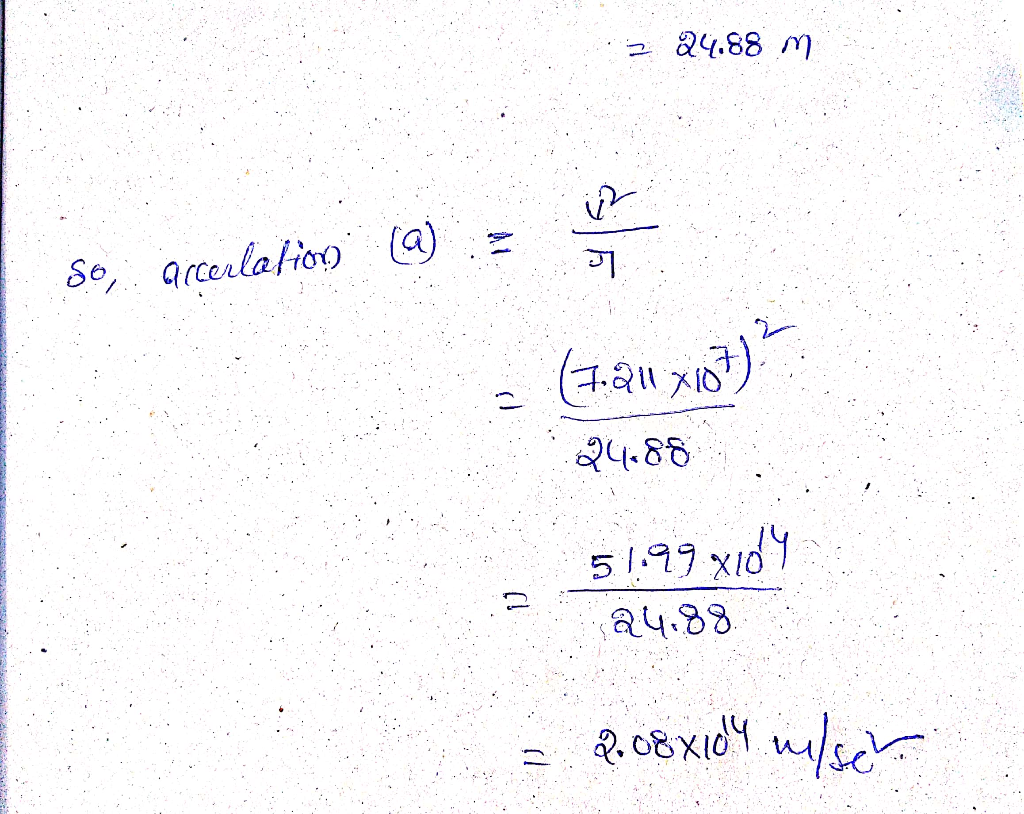#### Earn Coins

Coins can be redeemed for fabulous gifts.

Similar Homework Help Questions
• ### Your answer is partially correct. The electrons in the beam of a television tube have a...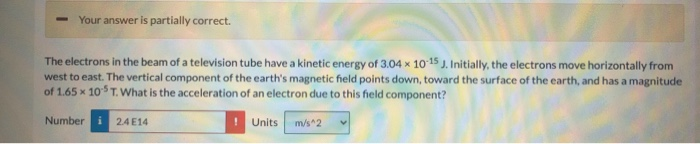Your answer is partially correct. The electrons in the beam of a television tube have a kinetic energy of 3.04 * 10-15 J. Initially, the electrons move horizontally from west to east. The vertical component of the earth's magnetic field points down, toward the surface of the earth, and has a magnitude of 1.65 x 10-ST. What is the acceleration of an electron due to this held component? Number 24 E14 Units m/s^2

• ### please show explanation! thank you Each of the electrons in the beam of a television tube...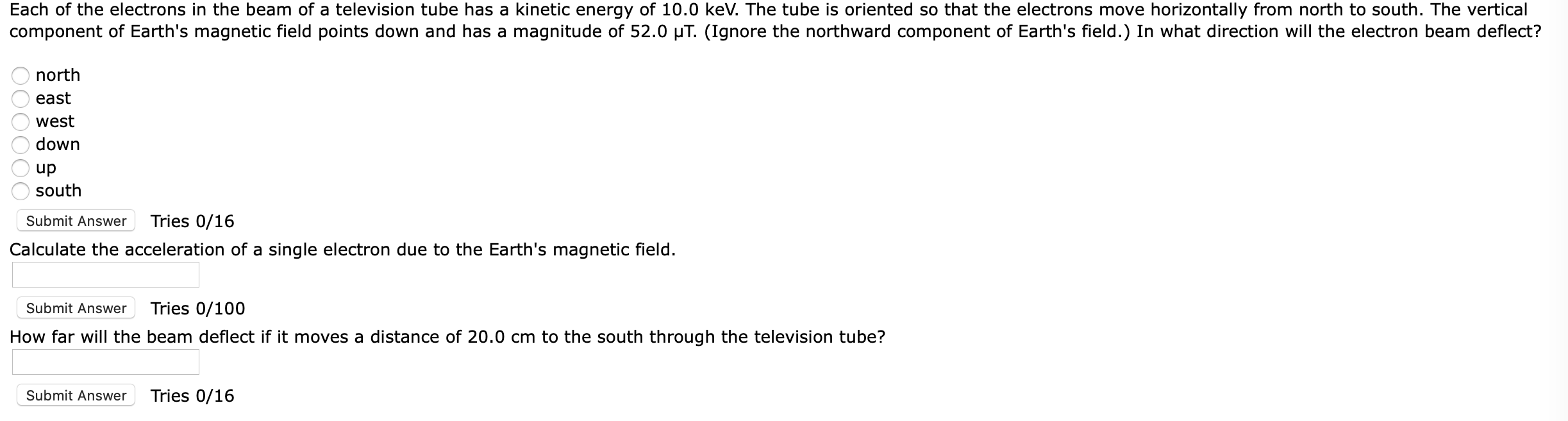please show explanation! thank you Each of the electrons in the beam of a television tube has a kinetic energy of 10.0 keV. The tube is oriented so that the electrons move horizontally from north to south. The vertical component of Earth's magnetic field points down and has a magnitude of 52.0 ut. (Ignore the northward component of Earth's field.) In what direction will the electron beam deflect? O north O east O west o down O up south Submit...

• ### Can you please show all work and calculations. Also can you explain how you got the...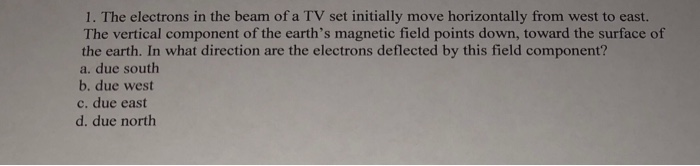Can you please show all work and calculations. Also can you explain how you got the answer! Thank you so much! 1·The electrons in the beam of a TV set initially move horizontally from west to east. The vertical component of the earth's magnetic field points down, toward the surface of the earth. In what direction are the electrons deflected by this field component? a. due south b. due west c. due east d. due north

• ### Q 11) An electron beam moves toward a cathode ray tube screen, which is 20 cm...

Q 11) An electron beam moves toward a cathode ray tube screen, which is 20 cm away from the negative electrode. The electrons are accelerated by a potential difference of 18 kV. Estimate the maximum displacement of the electron beam caused by Earth's magnetic field. The average magnetic field at the surface of Earth is roughly BE = 45×10^−6T. Express your answer with the appropriate units.

• ### The electron that flies in the tube has energy of 12 keV. The cathode-ray tube is oriented so that the electrons move in a horizontal direction from the south to the north. Earth's magnetic field...

The electron that flies in the tube has energy of 12 keV. The cathode-ray tube is oriented so that the electrons move in a horizontal direction from the south to the north. Earth's magnetic field component B = 5.5 * 10-5T is facing downwards. In what direction will the electron beam be tilted? How big is the electron beam deflection x when the electrons in the tube have flown 20 cm?

• ### An electron travels west to east with a kinetic energy of 10 keV. The Earth's magnetic...

An electron travels west to east with a kinetic energy of 10 keV. The Earth's magnetic field in Pittsburgh is 19,911.5 nT in the north direction, 3,257.1 nT in the west direction and 48,381.8 nT in the vertical direction. a) What is the force acting on the electron? b) What is the acceleration of the electron?

• ### The electron beam inside an old television picture tube is 0.40 mm in diameter and carries...

The electron beam inside an old television picture tube is 0.40 mm in diameter and carries a current of 50 μA. This electron beam impinges on the inside of the picture tube screen. A) How many electrons strike the screen each second? B) What is the current density in the electron beam? C) The electrons move with a velocity of 3.2 ×10^7 m/s. What electric field strength is needed to accelerate electrons from rest to this velocity in a distance...

• ### Each of the electrons in a particle beam has a kinetic energy of 1.36 ✕ 10−17...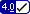Each of the electrons in a particle beam has a kinetic energy of 1.36 ✕ 10−17 J. The mass of an electron is 9.11 ✕ 10−31 kg. (a) What is the magnitude of the uniform electric field (pointing in the direction of the electrons' movement) that will stop these electrons in a distance of 11.0 cm?   N/C (b) How long will it take to stop the electrons?   ns (c) After the electrons stop, the electric field will continue to act...

• ### Each of the electrons in a particle beam has a kinetic energy of 1.42 ✕ 10−17...

Each of the electrons in a particle beam has a kinetic energy of 1.42 ✕ 10−17 J. The mass of an electron is 9.11 ✕ 10−31 kg. (a) What is the magnitude of the uniform electric field (pointing in the direction of the electrons' movement) that will stop these electrons in a distance of 14.0 cm? (b) How long will it take to stop the electrons? (c) After the electrons stop, the electric field will continue to act on them,...

• ### An electron with kinetic energy 228 eV is moving in a horizontal direction. The electron moves...

An electron with kinetic energy 228 eV is moving in a horizontal direction. The electron moves into a region where there is a uniform vertical electric field which has magnitude 3990 N/C. Find the smallest magnitude of a magnetic field that will cause the electron to continue to move horizontally. (1eV=1.6^10^(-19)J;mass of the electron=9.11*10^(-31)kg)